### Trigonometry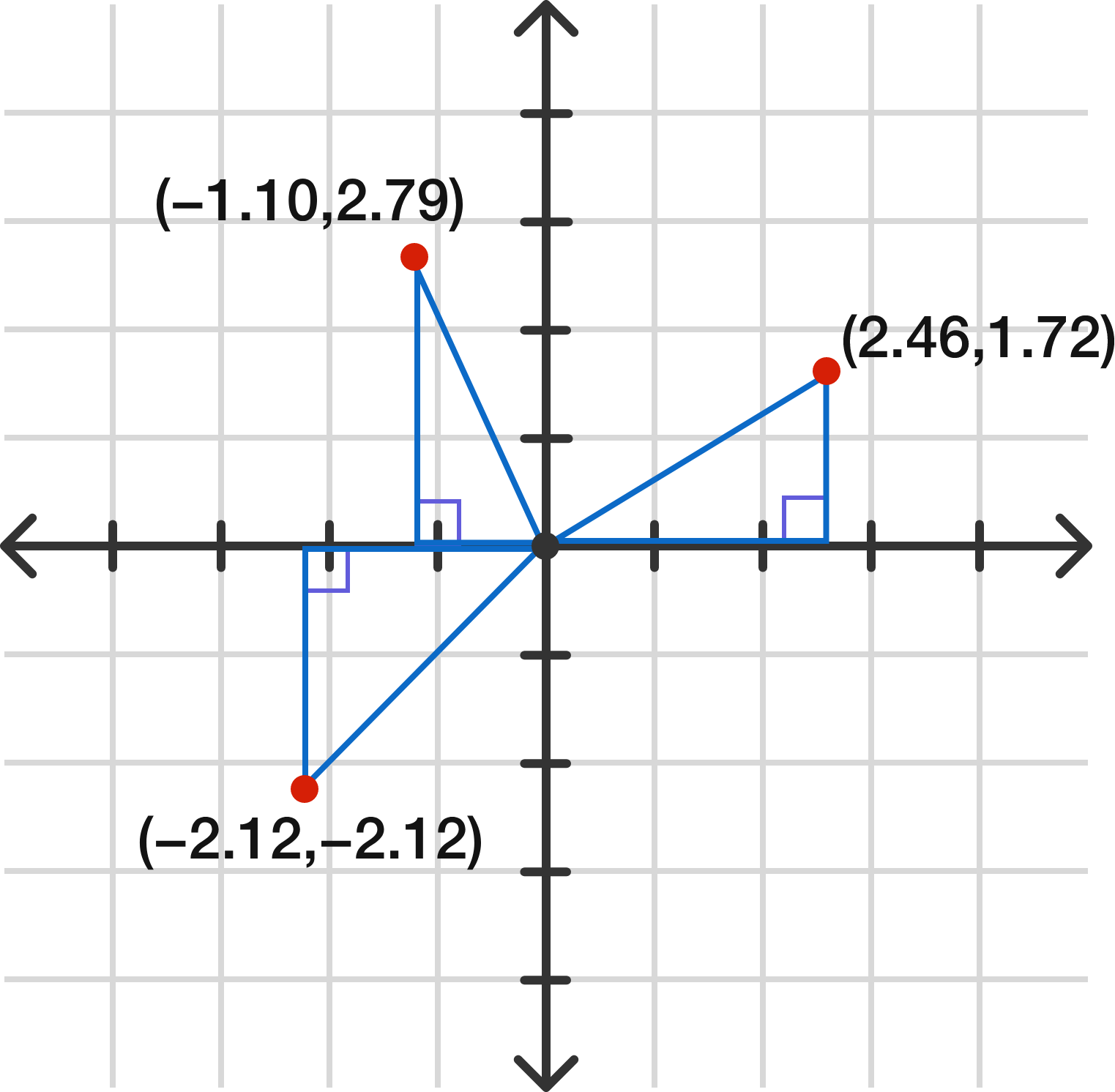The graph $x^2 + y^2 = 9$ can be considered the set of all solutions to the Pythagorean theorem $a^2 + b^2 = c^2,$ where $c$ is set at $3.$ Several points on the graph (with approximations) are shown above. Also, note that

\begin{aligned} (-1.10)^2 + (2.79)^2 &\approx 9 \\ (2.46)^2 + (1.72)^2 &\approx 9 \\ (-2.12)^2 + (-2.12)^2 &\approx 9. \end{aligned}

What does the entire graph of $x^2 + y^2 = 9$ look like?

# Thinking in Polar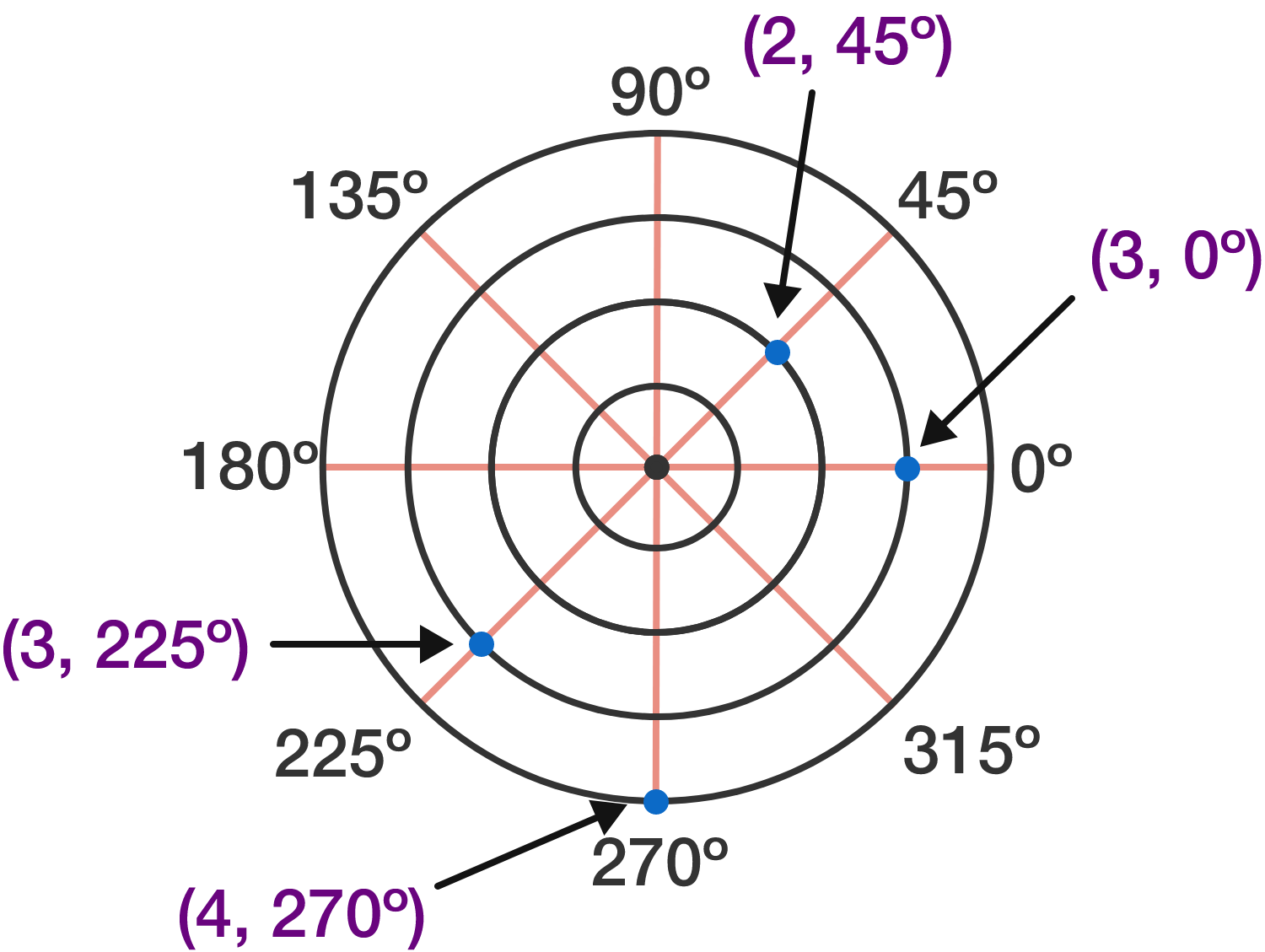In a traditional $xy$-coordinate system from the previous problem, the first value $x$ indicates horizontal distance and the second value $y$ indicates vertical distance. In a polar coordinate system using $(r, \theta),$ the value $r$ gives "distance from the origin when facing angle $\theta.$" Some example points are shown above.

One curious thing is that $r$ can be negative. Which point is $(-3, 135^\circ) ?$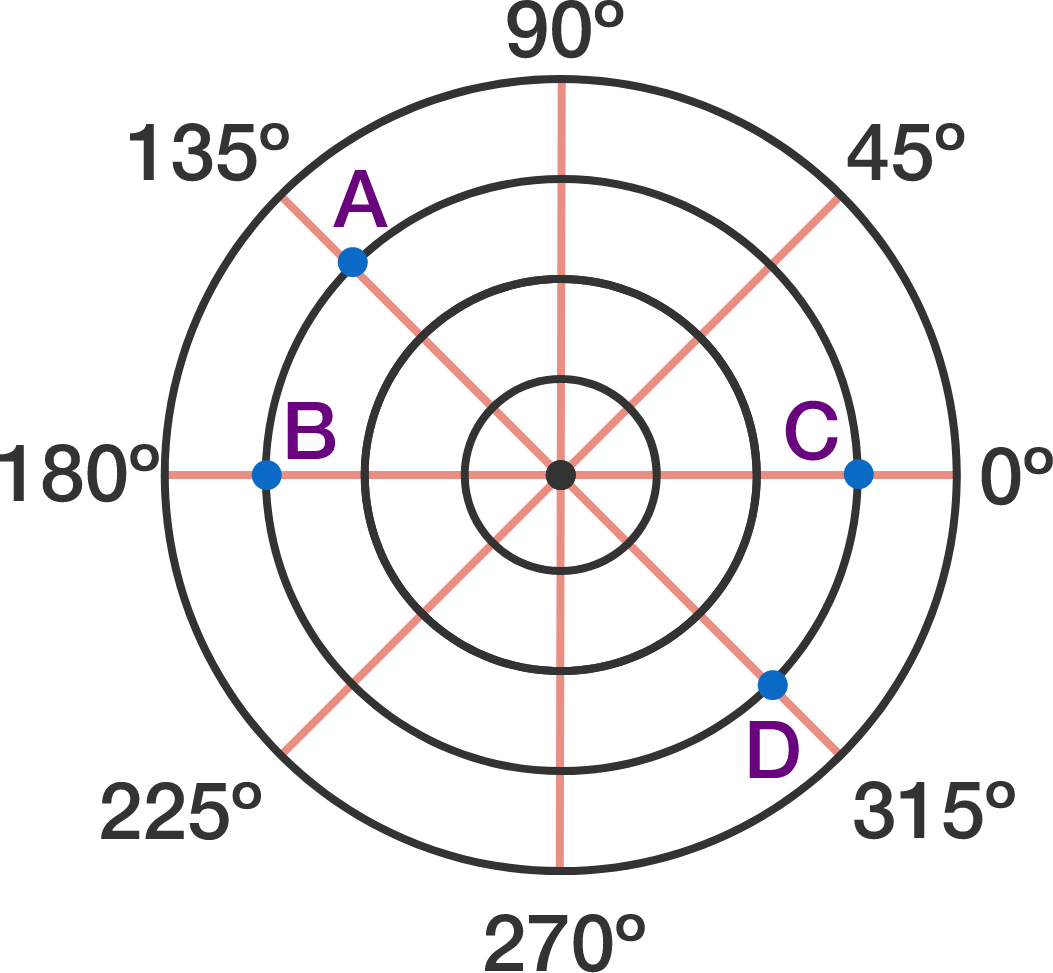# Thinking in Polar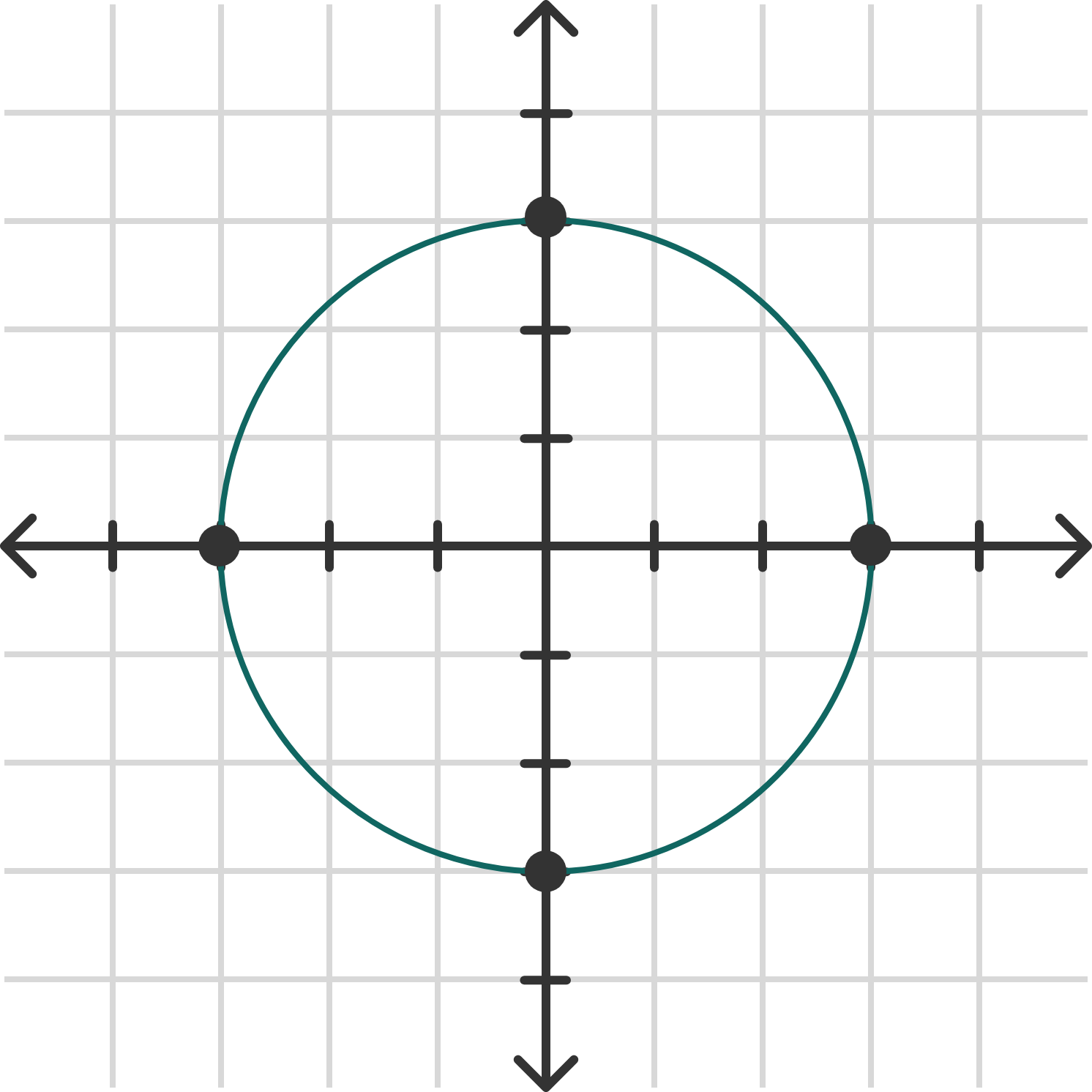We saw the graph of the circle above written as $x^2 + y^2 = 9$ in rectangular coordinates. How can you write it using polar coordinates?

# Thinking in Polar

Other than the same point being identified with a positive $r$ (facing forward) or a negative $r$ (facing backward), it's possible for the same point to be identified in an infinite number of ways via the measure of angle.

The point below can be identified as $(2, 180^\circ) .$ Which of the choices also identifies the same point?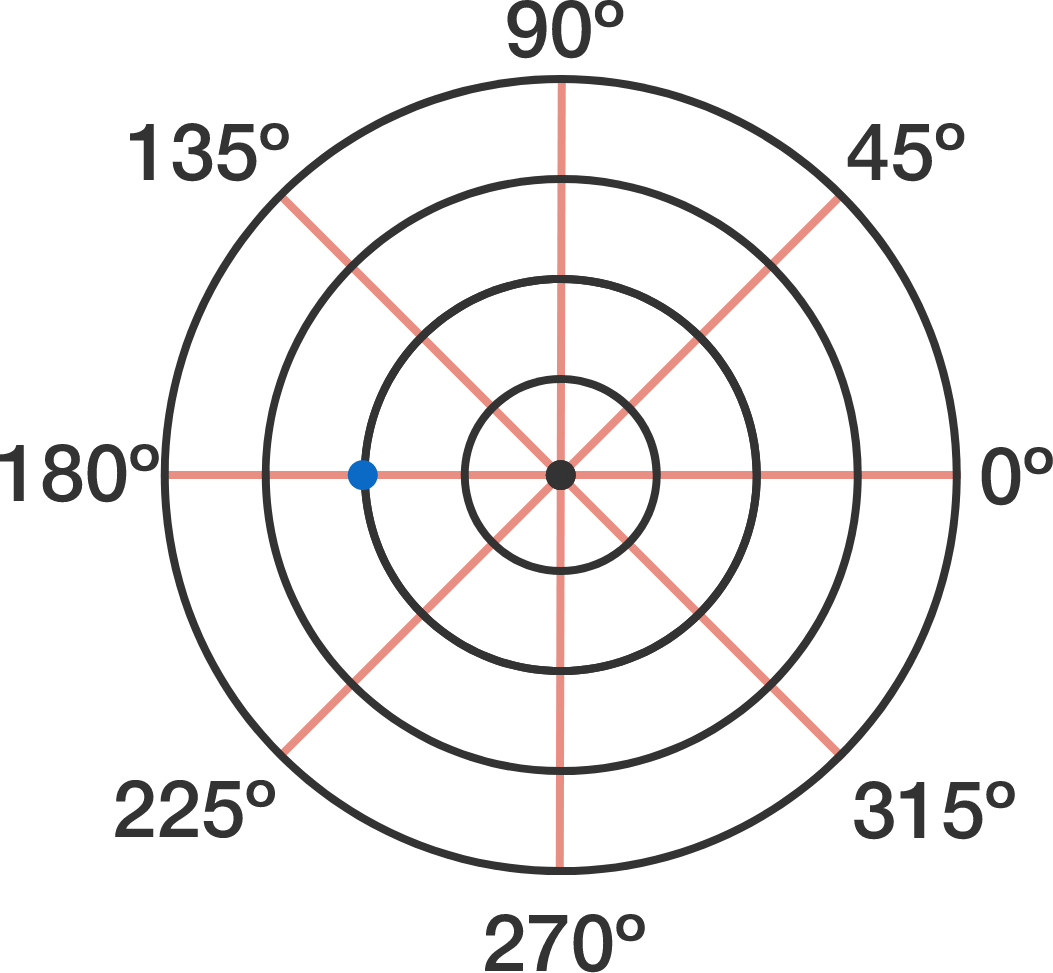# Thinking in Polar

In the visualization above, $r$ is restricted to only negative values. It can still be used to help to answer the question "Which pair of coordinates refer to the same point?"

# Thinking in Polar

Because of possible negative values, polar graphs are often restricted to particular values of $r$ and $\theta .$

If we restrict $\theta$ to positive values only, what kind of graph will $r= \theta$ be?

# Thinking in Polar

The visualization below lets you see the effect of $b$ in the spiral graph $r = b \theta .$

What will happen to the graph if $b$ is allowed to be negative?

# Thinking in Polar

Polar coordinates give the flexibility to include whole new classes of graphs, like the flower with overlapping petals above. Many other marvels await: start now with an intuitive look at the six main functions of trigonometry.

×